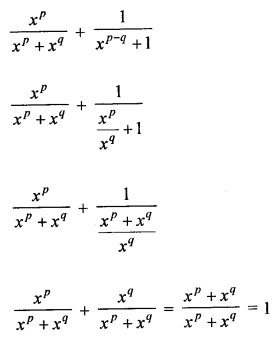## ML Aggarwal Class 8 Solutions for ICSE Maths Chapter 2 Exponents and Powers Objective Type Questions

Mental Maths

Question 1.
Fill in the blanks:
(i) Multiplicative inverse of $$\left( \frac { -4 }{ 5 } \right) ^{ 2 }$$ = ……..
(ii) The standard form of 0.000182 = ………
(iii) The standard form of ……….. is 0.00000003572.
(iv) Expanded form of 273.92 = ………..
(v) If (-7)x × $$\left( \frac { 1 }{ -7 } \right) ^{ -3 }$$ = 78, then value of x is …….
(vi) If 3n = 729, then n = ……
(vii) The value of (70 + 50) is ……..
Solution:
(i) Multiplicative inverse of $$\left( \frac { -4 }{ 5 } \right) ^{ 2 }$$ or $$\frac { 16 }{ 25 }$$ is $$\frac { 25 }{ 16 }$$
(ii) The standard form of 0.000182 = 1.82 × 10-4.
(iii) The standard form of 3.572 × 10-8 is 0.00000003572.
(iv) Expanded form of 273.92 = 2 × 102 + 7 × 10 + 3 × 100 + 9 × 10-1 + 2 × 10-2.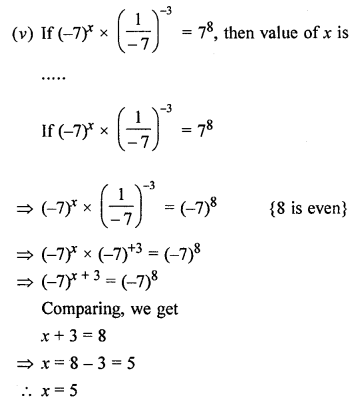(vii) The value of (70 + 50) is 1 + 1 = 2.

Question 2.
State whether the following statements are true (T) or false (F):
(i) The value of (-3)-2 is $$\frac { 1 }{ -9 }$$.
(ii) For negative integers m and n, am × an = am+n (a ≠ 0).
(iii) 30 × 40 = 120.
(iv) The value of 37 – 27 is 17.
(v) The standard form of 0.0034 is 3.4 × 103.
(vi) Multiplicative inverse of (-2)5 is $$\frac { 1 }{ -32 }$$.
Solution:
(i) The value of (-3)-2 is $$\frac { 1 }{ -9 }$$. (False)
(ii) For negative integers m and n, am × an = am+n (a ≠ 0). (True)
(iii) 30 × 40 = 120. (True)
⇒ 1 × 1 = 1 (a0 = 1)
(iv) The value of 37 – 2is 17. (False)
Correct:
37 – 27 = 3 × 3 × 3 ………. 7 times – 2 × 2 × 2 …. 7 times ≠ 1
But 17 = 1
(v) The standard form of 0.0034 is 3.4 × 103. (True)
(vi) Multiplicative inverse of (-2)5 is $$\frac { 1 }{ -32 }$$. (True)
Multiplicative inverse of (-2)5 = (-2) × (-2) × (-2) × (-2) × (-2) × ……… = -32
Inverse = $$\frac { 1 }{ -32 }$$.

Multiple Choice Questions

Choose the correct answer from the given four options (3 to 12):
Question 3.
$$\left( \frac { 1 }{ 2 } \right) ^{ -5 }$$ is equal to
(a) 32
(b) $$\frac { 1 }{ 32 }$$
(c) -32
(d) $$\frac { -1 }{ 32 }$$
Solution:
$$\left( \frac { 1 }{ 2 } \right) ^{ -5 }$$ = (2)5 = 2 × 2 × 2 × 2 × 2 = 32 (a)

Question 4.Solution: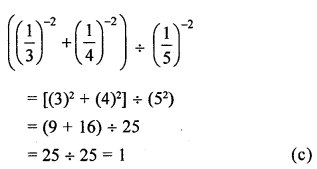Question 5.
If 24 × 43 = 4x, then the value of x is
(a) 5
(b) 7
(c) 4
(d) -5
Solution:
24 × 43 = 4x
⇒ 24 × (22)3 = (22)x
⇒ 24 × 26 = 22x
⇒ 210 = 22x
2x = 10
⇒ x = 5 (a)

Question 6.Solution: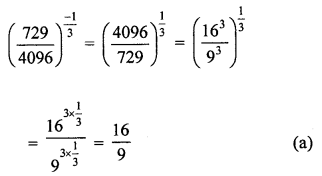Question 7.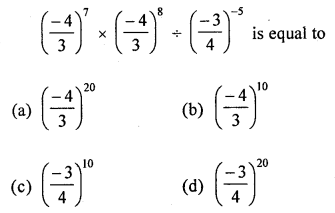Solution: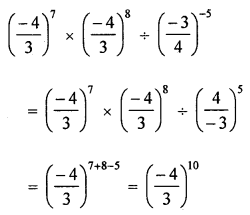Question 8.
Standard form of 0.000000789 is
(a) 78.9 × 10-8
(b) 789 × 10-9
(c) 7.89 × 10-7
(d) None
Solution:
0.000000789 = 7.89 × 10-7 (c)

Question 9.
The usual form of 3.67 × 105 is
(a) 3670000
(b) 367000
(c) 36700
(d) 3670
Solution:
3.67 × 105 = 367000

Question 10.
Multiplicative inverse of $$\left( \frac { -2 }{ 3 } \right) ^{ 4 }$$ is
(a) $$\frac { 16 }{ 81 }$$
(b) $$\frac { -16 }{ 81 }$$
(c) $$\frac { 81 }{ 16 }$$
(d) $$\frac { -81 }{ 16 }$$
Solution:Question 11.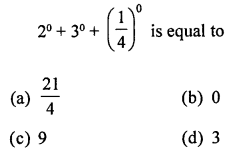Solution: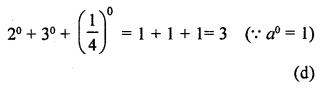Question 12.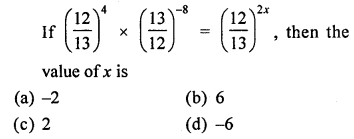Solution: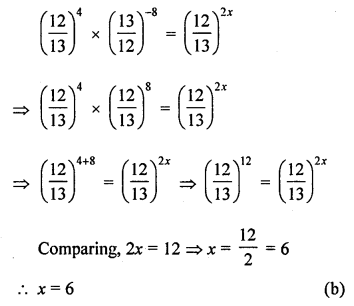Value Based Questions

Question 1.
Anaemia causes due to deficiency of Red Blood cells. The size of a red blood cell is 0.000000007 mm. Express it in standard form. Rahul is suffering from anemia, his doctor adviced him to take healthy food regularly and avoid junk food.
Why should we eat healthy food? What values are being promoted?
Solution:
Size of a red blood cell = 0.000000007 mm = 7.0 × 10-9 mm
Deficiency of red blood cells causes anaemia so, in order to keep healthy,
healthy food should be taken and avoid junk food.

Higher Order Thinking Skills (HOTS)

Question 1.
Find the multiplicative inverse of (50 + 30) (50 – 30).
Solution:
(50 + 30) (50 – 30) = (1 + 1) (1 – 1) = 2 × 0 = 0
Multiplicative inverse of 0 does not exist.

Question 2.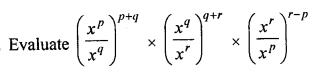Solution:Question 3.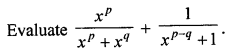Solution: